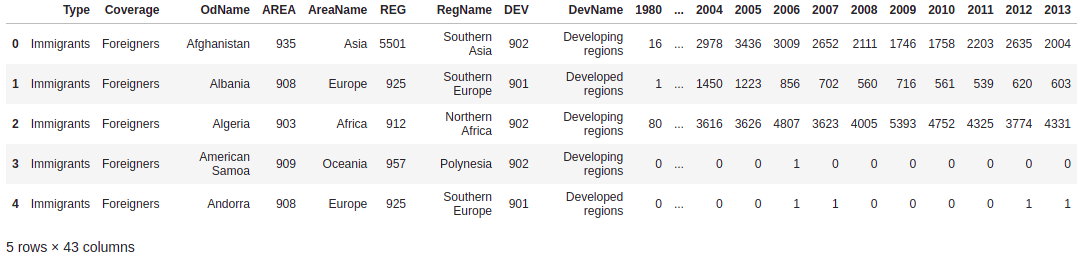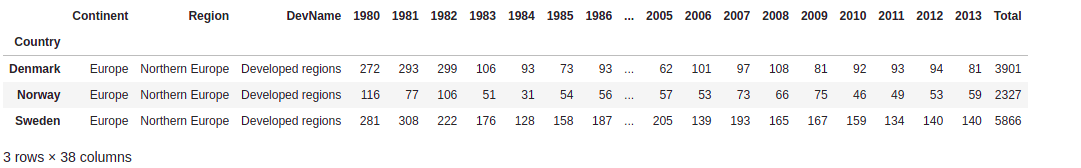## Accelerators

### Go to Overview

KDP KDSP

PremonR Studio9

#### TechHub

Akka Scala Rust Spark Functional Java Kafka Flink ML/AI DevOps Data Warehouse

## Insights# Waffle Chart

A waffle chart is an interesting visualization that is normally created to display progress toward goals. It is commonly an effective option when you are trying to add interesting visualization features to a visual that consists mainly of cells, such as an Excel dashboard. so in this blog we will learn how to create waffle chart using python.

If you want to go through some ML concepts here is the link for you.

so, let’s get started

first We will import the modules

``````!pip install openpyxl
import numpy as np  # useful for many scientific computing in Python
import pandas as pd # primary data structure library
from PIL import Image # converting images into arrays
import openpyxl
``````

now, we will import our dataset

``````df_can = pd.read_excel(
skiprows=range(20),
skipfooter=2)

###### perform some basic operation
``````df_can.head()
``````Let’s find out how many entries there are in our dataset
``````# print the dimensions of the dataframe
print(df_can.shape)

``````

output: (195,43)

``````# clean up the dataset to remove unnecessary columns (eg. REG)
df_can.drop(['AREA','REG','DEV','Type','Coverage'], axis = 1, inplace = True)

# let's rename the columns so that they make sense
df_can.rename (columns = {'OdName':'Country', 'AreaName':'Continent','RegName':'Region'}, inplace = True)

# for sake of consistency, let's also make all column labels of type string
df_can.columns = list(map(str, df_can.columns))

# set the country name as index - useful for quickly looking up countries using .loc method
df_can.set_index('Country', inplace = True)

df_can['Total'] =  df_can.sum (axis = 1)

# years that we will be using in this lesson - useful for plotting later on
years = list(map(str, range(1980, 2014)))
print ('data dimensions:', df_can.shape)
``````
```output: data dimensions: (195, 38)
```

### Visualizing Data using Matplotlib

``````%matplotlib inline

import matplotlib as mpl
import matplotlib.pyplot as plt
import matplotlib.patches as mpatches # needed for waffle Charts

mpl.style.use('ggplot') # optional: for ggplot-like style

print ('Matplotlib version: ', mpl.__version__) # >= 2.0.0

``````

#### Waffle Charts

``````# let's create a new dataframe for these three countries
df_dsn = df_can.loc[['Denmark', 'Norway', 'Sweden'], :]

# let's take a look at our dataframe
df_dsn
``````Step 1. The first step into creating a waffle chart is determining the proportion of each category with respect to the total.
```# compute the proportion of each category with respect to the total
total_values = df_dsn['Total'].sum()
category_proportions = df_dsn['Total'] / total_values

# print out proportions
pd.DataFrame({"Category Proportion": category_proportions})```Step 2. The second step is defining the overall size of the waffle chart.
``````width = 40 # width of chart
height = 10 # height of chart

total_num_tiles = width * height # total number of tiles

print(f'Total number of tiles is {total_num_tiles}.')``````

output:

```Total number of tiles is 400.

```

Step 3. The third step is using the proportion of each category to determine it a respective number of tiles .

``````# compute the number of tiles for each category
tiles_per_category = (category_proportions * total_num_tiles).round().astype(int)

# print out number of tiles per category
pd.DataFrame({"Number of tiles": tiles_per_category})
``````

Based on the calculated proportions, Denmark will occupy 129 tiles of the `waffle` chart, Norway will occupy 77 tiles, and Sweden will occupy 194 tiles.

Step 4. The fourth step is creating a matrix that resembles the `waffle` chart and populating it.

``````# initialize the waffle chart as an empty matrix
waffle_chart = np.zeros((height, width), dtype = np.uint)

# define indices to loop through waffle chart
category_index = 0
tile_index = 0

# populate the waffle chart
for col in range(width):
for row in range(height):
tile_index += 1

# if the number of tiles populated for the current category is equal to its corresponding allocated tiles...
if tile_index > sum(tiles_per_category[0:category_index]):
# ...proceed to the next category
category_index += 1

# set the class value to an integer, which increases with class
waffle_chart[row, col] = category_index

print ('Waffle chart populated!')
``````

output:

```Waffle chart populated!
```

Let’s take a peek at how the matrix looks like.

``````waffle_chart

``````

As expected, the matrix consists of three categories and the total number of each category’s instances matches the total number of tiles allocated to each category.

Step 5. Map the `waffle` chart matrix into a visual.

``````# instantiate a new figure object
fig = plt.figure()

# use matshow to display the waffle chart
colormap = plt.cm.coolwarm
plt.matshow(waffle_chart, cmap=colormap)
plt.colorbar()
plt.show()
``````

Step 6. Prettify the chart.

``````# instantiate a new figure object
fig = plt.figure()

# use matshow to display the waffle chart
colormap = plt.cm.coolwarm
plt.matshow(waffle_chart, cmap=colormap)
plt.colorbar()

# get the axis
ax = plt.gca()

# set minor ticks
ax.set_xticks(np.arange(-.5, (width), 1), minor=True)
ax.set_yticks(np.arange(-.5, (height), 1), minor=True)

# add gridlines based on minor ticks
ax.grid(which='minor', color='w', linestyle='-', linewidth=2)

plt.xticks([])
plt.yticks([])
plt.show()
``````

### Conclusion

in this blog we have learned how to draw a waffle chart for provided dataset as it is an interesting visualization that is normally created to display progress toward goals. For more details you visit this link.#### Written by Lokesh Kumar

Lokesh Kumar is intern in AI/ML studio at Knoldus. He is passionate about Artificial Intelligence and Machine Learning , having knowledge of C , C++ , Python and Data Analytics and much more. He is recognised as a good team player, a dedicated and responsible professional, and a technology enthusiast. He is a quick learner & curious to learn new technologies.